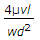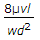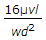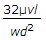# Mechanical Engineering - Hydraulics and Fluid Mechanics

### Exercise :: Hydraulics and Fluid Mechanics - Section 6

31.

The loss of head due to viscosity for laminar flow in pipes is (where d = Diameter of pipe, l = Length of pipe, v = Velocity of the liquid in the pipe, μ = Viscosity of the liquid, and w = Specific weight of the flowing liquid)

 A.B.C.D.Answer: Option D

Explanation:

No answer description available for this question. Let us discuss.

32.

The viscosity of water at 20°C is

 A. one stoke B. one centistroke C. one poise D. one centipoise

Answer: Option D

Explanation:

No answer description available for this question. Let us discuss.

33.

The rise of liquid along the walls of a revolving cylinder about the initial level is __________ the depression of the liquid at the axis of rotation.

 A. same as B. less than C. more than

Answer: Option A

Explanation:

No answer description available for this question. Let us discuss.

34.

The discharge through a channel of rectangular section will be maximum, if

 A. its depth is twice the breadth B. its breadth is twice the depth C. its depth is thrice the breadth D. its breadth is thrice the depth

Answer: Option B

Explanation:

No answer description available for this question. Let us discuss.

35.

When a body, floating in a liquid, is given a small angular displacement, it starts oscillating about a point known as

 A. centre of pressure B. centre of gravity C. centre of buoyancy D. metacentre

Answer: Option D

Explanation:

No answer description available for this question. Let us discuss.

#### Current Affairs 2021

Interview Questions and Answers